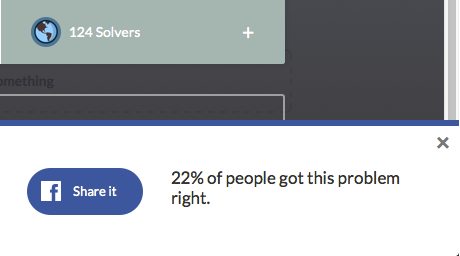# Brilliant and Problem Solvers

Algebra Level 3The above picture shows (as of 12/15/14) the number of solvers of the first problem I posted on Brilliant, Yay for 2014! #1. The percentage in the box below the number of solvers is the percentage of viewers who solved the problem.

Now, this percentage isn't an exact figure; in fact, if the exact percentage of viewers that solved the problem is $p\%,$ then the percentage that appears in the box is $\left \lfloor p \right \rfloor \%.$ For example, if the number of viewers on a particular problem is 7, and 3 of those viewers solve it, the percentage in the box would be $42\%,$ when in fact the exact percentage is $42\frac{6}{7}\%.$

Given this, the sum of all the possible number of viewers that could possibly have viewed my problem is equal to $N.$ Find the last two digits of $N.$

Notation: $\lfloor \cdot \rfloor$ denotes the floor function.

×

Problem Loading...

Note Loading...

Set Loading...